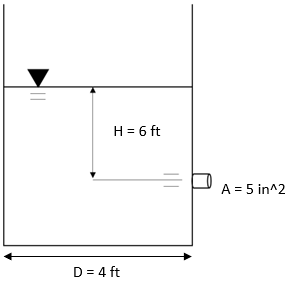## Time to Drain

The figure shown is a cross sectional view of a circular water tank with a 4 ft diameter that discharges water freely into the atmosphere. If the output nozzle has a 5 in^2 cross-sectional area, what is the time (in seconds) required to drain the water line from 6 ft to 2 ft? Assume no minor losses in discharge.Hint
The time required to drain a circular tank:
$$\Delta t=\frac{2(A_t/A_0)}{\sqrt{2g}}(h_{1}^{0.5}-h_{2}^{0.5})$$$where $$A_t$$ is the tank’s cross-sectional area, $$A_0$$ is the cross-sectional area of fluid flow, $$g$$ is the acceleration due to gravity, $$h_1$$ is the initial fluid line height, and $$h_2$$ is the final fluid line height. Hint 2 $$A_t=\frac{\pi D_{t}^{2}}{4}$$$
where $$D_t$$ is the circular tank’s diameter.
First, let’s find the tank’s cross-sectional area:
$$A_t=\frac{\pi D_{t}^{2}}{4}$$$where $$D_t$$ is the circular tank’s diameter. $$A_t=\frac{\pi (4ft)^{2}}{4}=12.566\:ft^2$$$
The time required to drain a circular tank:
$$\Delta t=\frac{2(A_t/A_0)}{\sqrt{2g}}(h_{1}^{0.5}-h_{2}^{0.5})$$$where $$A_t$$ is the tank’s cross-sectional area, $$A_0$$ is the cross-sectional area of fluid flow, $$g$$ is the acceleration due to gravity, $$h_1$$ is the initial fluid line height, and $$h_2$$ is the final fluid line height. $$\Delta t=\frac{2(12.57ft^2/(5\div 12\div 12ft^2))}{\sqrt{2(32.2ft/s^2)}}((6ft)^{0.5}-(2ft)^{0.5})$$$
$$\Delta t=\frac{2(12.57/0.0347)}{\sqrt{64.4ft/s^2}}(2.45\sqrt{ft}-1.41\sqrt{ft})$$$$$\Delta t=\frac{723.8}{8.02\frac{\sqrt{ft}}{s}}(1.04\sqrt{ft})=93.9s=94\:sec$$$
94 sec